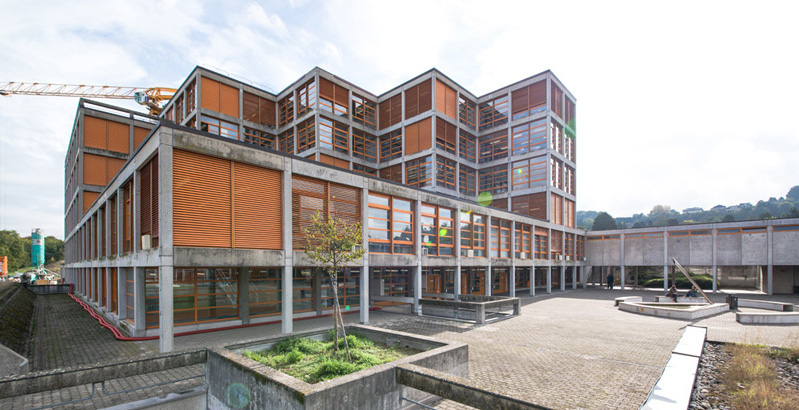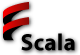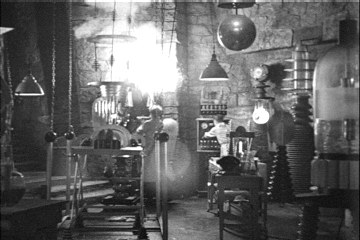# Programmation appliquée en ScalaCopyright © Cay S. Horstmann 2015# Why Scala?• Object-oriented and functional
• Statically typed
• Scalable from simple scripts to sophisticated (but still easy-to-use) libraries
• Invented by Martin Odersky at EPFL
• Works with Java VM tools/libraries/infrastructure
• Used for big-iron projects in industry
• Pragmatic choice as successor to Java (whose evolution has slowed to a crawl)
• Expands your mind and makes you think differently about programming in any language

# About the Course

• Meets Monday 13:00 - 16:25
• Lecture/Lab/Break/Lecture/Lab
• Short lectures/long labs
• You can ask questions/write reports in English or French
• Course/exams: 40%, labs/homeworks: 30%, project: 30%

# What is the Most Important Skill...

...that HEIG-VD computer science graduates wish they had learned better in college?

1. Object-Oriented Programming
2. Functional Programming
3. Programming Projects
4. Giving Presentations in English

# Do You Already Know Some Scala?

1. No
2. Just a little
3. I am reasonably fluent
4. I am an expert

# Scala Basics

• Types `Int`, `Double`, `Boolean`, `String`
• Arithmetic like in C++/Java: `+ - * / %`
• Variable type is inferred:
`val luckyNumber = 13 // luckyNumber is an Int`
• Function types:
`val square = (x: Int) => x * x // square is an Int => Int`
• Semicolons at the end of a line are optional
```val x = 1
val y = 2 + // end line with operator to indicate that there is more to come
3```
• Everything is an object
`1.to(10) // Apply to method to 1, returns Range(1, 2, 3, 4, 5, 6, 7, 8, 9, 10)`

# Map

• `1.to(10)` yields a `Range`
• Collections `List`, `Vector` (similar to C++), `Range`, many more
• `map` works on any collection
```val square = (x: Int) => x * x
1.to(9).map(square)
// Yields 1 4 9 16 25 ... 81```
• Applies function to all elements and yields collection of results
• What is
`1.to(9).map(square).map(x => x % 10).distinct`
• No `()` behind `distinct`. Rule of thumb: no args and not mutating ⇒ no `()`
• How many lines of code would this take in C++?

# Functional Programming

• Functional programming: Functions are values
• In C++, values are
• Primitive types `int`, `double`, etc.
• Structure/class values
• Pointers
• A function is not a “first class” value in C++
• Cannot create new functions in a running program
• In a functional programming language, functions are first-class values
• Can have variables that hold functions
• Can create new functions

# Functional Programming in Scala

• Functions can be values
```val num = 3.14
val fun = math.ceil _
fun(num) // prints 4```
• Functions can be anonymous...
`(x: Int) => x * x`
• ...just like numbers
`3.14`
• Of course, can put function values into variables and then use them
```val square = (x: Int) => x * x
square(10) // prints 100```
• ...again, just like numbers
```val pi = 3.14
pi * 10 // prints 31.4```

# Why Functional Programming?

• Simpler and clearer programming style
• Often useful to pass functions as parameters
```val numbers = Vector(1, 2, 3, 4, 5, 6, 7, 8, 9, 10)
numbers.map((x: Int) => x * x)
// Prints Vector(1, 4, 9, 16, 25, 36, 49, 64, 81, 100)```
• In C++, you can also do this, but there are no anonymous functions.
```vector<int> map(vector<int> values, int (*f)(int)) {
vector<int> result;
for (int i = 0; i < size; i++) result.push_back(f(values[i]));
return result;
}
int square(int x) { return x * x; }
...
res = map(numbers, square); // Must pass name of function```
• In Scala, but not in C++, a function can produce a new function

# Immutability

• Immutable: Cannot change
• In Java, strings are immutable
• `"Hello".toUpper()` doesn't change `"Hello"` but returns a new string `"HELLO"`
• In Scala, `val` are immutable
```val num = 3.14
num = 1.42 // Error```
• Pure functional programming: No mutations
• Don't mutate—always return the result as a new value
• Functions that don't mutate state are inherently parallelizable
• Important consideration in light of the end of Moore's Law

# Lab• Format of classes: approx. 20 minutes lecture, 40 minutes lab, 15 minutes wrap-up
• You work with a buddy
• One of you (the coder) writes the code, the other (the scribe) types up answers
• When you get stuck, ask your buddy first!
• Switch coder/scribe roles each lab
• The coder submits worksheet to Fatemeh.Borran@heig-vd.ch. Include the scribe's name in the worksheet!
• The scribe submits answers to Fatemeh.Borran@heig-vd.ch. Include the coder's name in the report!

# Part 1: The Scala Worksheet

1. Start the Scala IDE and make a new Scala project: File -> New -> Scala Project. Give a name `Worksheets`. Right-click on the project in the Package Explorer, then select New -> Scala Worksheet. Call it `Day1`.
2. Make a new line before the `}` . Type `6 * 7` and then save (Ctrl+S/⌘+S). What do you get?
3. Edit the line to read `val a = 6 * 7` and save. What do you get?
4. Add `a`. What do you get?
5. Add `a = 43`. What do you get? Why?
6. Remove that line and add `val b;` (This time with a semicolon.) What do you get? Why?
7. Now remove that line.

# Part 2: Functions are Values

1. Add `val triple = (x: Int) => 3 * x`. What do you get?
2. Add `triple(5)`. What do you get?

Tip: These “What do you get” exercises are a lot more enjoyable and effective when you and your buddy first discuss what you think you'll get before you execute the Save command.

3. Add `triple`. What do you get?
4. What is the type of `triple` in Scala?
5. What is the type of `5` in Scala?

# Part 3: Functions as Parameters

1. Type `1.to(10)`. What do you get?
2. Type `1.to(10).map(triple)`. What do you get? Why?
3. How do you get the cubes of the numbers from 1 to 10?
4. How do you get the cubes of the numbers from 1 to 10 without using `val`? Hint: Anonymous functions

# Part 4: Quadratic Residues (Extra Credit)

1. Do you remember this?

`0.to(9).map(square).map(x => x % 10).distinct`

Try it out. What did you get?

2. These are called the “quadratic residues modulo 10”—the values that are squares of some value in { 0...9 }.
3. What are the quadratic residues modulo 11? Modulo 16?
4. Modulo `n`? Write a function `val qres = (n: Int) => ... ` that produces them. What is your function? What is `qres(20)`?
5. For `n` going from 2 to 20, how many quadratic residues are there?

Hint: `2.to(20).map(...)` and `map(l -> l.length)`

6. Did you get it right? Check against the table in this page. In that table, 0 is not considered a quadratic residue, so your counts should be one higher.
7. What would it have taken to do this in C++?